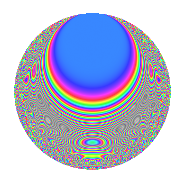# Properties

 Label 4031.2.a.cLevel 4031 Weight 2 Character orbit 4031.a Self dual yes Analytic conductor 32.188 Analytic rank 1 Dimension 61 CM no Inner twists 1

# Related objects

## Newspace parameters

 Level: $$N$$ = $$4031 = 29 \cdot 139$$ Weight: $$k$$ = $$2$$ Character orbit: $$[\chi]$$ = 4031.a (trivial)

## Newform invariants

 Self dual: yes Analytic conductor: $$32.1876970548$$ Analytic rank: $$1$$ Dimension: $$61$$ Coefficient ring index: multiple of None Twist minimal: yes Fricke sign: $$1$$ Sato-Tate group: $\mathrm{SU}(2)$

## $q$-expansion

The dimension is sufficiently large that we do not compute an algebraic $$q$$-expansion, but we have computed the trace expansion.

 $$\operatorname{Tr}(f)(q) =$$ $$61q - q^{2} - 4q^{3} + 43q^{4} - 7q^{5} - 13q^{6} - 10q^{7} - 6q^{8} + 23q^{9} + O(q^{10})$$ $$\operatorname{Tr}(f)(q) =$$ $$61q - q^{2} - 4q^{3} + 43q^{4} - 7q^{5} - 13q^{6} - 10q^{7} - 6q^{8} + 23q^{9} - 16q^{10} - 3q^{11} - 18q^{12} - 28q^{13} - 14q^{14} - 12q^{15} + 11q^{16} - 21q^{17} - 17q^{18} - 36q^{19} - 16q^{20} - 12q^{21} - 42q^{22} - 15q^{23} - 28q^{24} - 16q^{25} - 13q^{26} - 10q^{27} - 25q^{28} - 61q^{29} - 12q^{30} - 18q^{31} - 3q^{32} - 42q^{33} - 22q^{34} - 29q^{35} - 38q^{36} - 30q^{37} - 27q^{38} - 31q^{39} - 22q^{40} - 28q^{41} - 9q^{42} - 58q^{43} - 2q^{44} - 31q^{45} - 40q^{46} - 6q^{47} - 37q^{48} - 37q^{49} - 15q^{50} - 44q^{51} - 43q^{52} - 27q^{53} - 18q^{54} - 38q^{55} - 22q^{56} - 50q^{57} + q^{58} - 24q^{59} + 6q^{60} - 76q^{61} - 17q^{62} - 6q^{63} - 60q^{64} - 65q^{65} - 7q^{66} - 45q^{67} - 31q^{68} - 16q^{69} - 48q^{70} - 28q^{71} - 40q^{72} - 50q^{73} - 17q^{74} - 35q^{75} - 100q^{76} + q^{77} - 6q^{78} - 66q^{79} - 10q^{80} - 63q^{81} - 5q^{82} - 9q^{83} - 24q^{84} - 77q^{85} + 29q^{86} + 4q^{87} - 62q^{88} - 30q^{89} + 50q^{90} - 52q^{91} - 53q^{92} - 42q^{93} - 92q^{94} - 20q^{95} - 47q^{96} - 34q^{97} + 36q^{98} - 29q^{99} + O(q^{100})$$

## Embeddings

For each embedding $$\iota_m$$ of the coefficient field, the values $$\iota_m(a_n)$$ are shown below.

For more information on an embedded modular form you can click on its label.

Label $$a_{2}$$ $$a_{3}$$ $$a_{4}$$ $$a_{5}$$ $$a_{6}$$ $$a_{7}$$ $$a_{8}$$ $$a_{9}$$ $$a_{10}$$
1.1 −2.62831 0.935172 4.90799 −2.36331 −2.45792 0.343928 −7.64309 −2.12545 6.21151
1.2 −2.62306 1.48834 4.88043 0.915377 −3.90400 −2.34390 −7.55555 −0.784847 −2.40109
1.3 −2.53428 −2.04027 4.42257 −2.47983 5.17061 −0.629929 −6.13948 1.16269 6.28458
1.4 −2.49843 −0.867725 4.24214 3.27534 2.16795 1.98132 −5.60183 −2.24705 −8.18321
1.5 −2.42000 −2.29529 3.85639 1.32254 5.55461 0.993943 −4.49245 2.26837 −3.20054
1.6 −2.31412 0.311751 3.35513 1.29585 −0.721429 −1.13716 −3.13593 −2.90281 −2.99874
1.7 −2.25610 3.35950 3.09001 −1.55616 −7.57937 −0.413231 −2.45917 8.28621 3.51085
1.8 −2.23009 1.62667 2.97329 2.06861 −3.62762 3.32810 −2.17052 −0.353941 −4.61317
1.9 −2.10281 −1.04272 2.42182 3.50696 2.19264 −3.91864 −0.887016 −1.91274 −7.37448
1.10 −1.99079 −3.03517 1.96324 −1.96489 6.04239 0.618316 0.0731745 6.21228 3.91168
1.11 −1.87723 1.73503 1.52398 −2.87174 −3.25705 −0.555991 0.893598 0.0103383 5.39090
1.12 −1.83395 2.22148 1.36337 1.24306 −4.07408 2.01007 1.16755 1.93497 −2.27970
1.13 −1.77273 −1.39503 1.14258 −2.16266 2.47302 3.73562 1.51997 −1.05388 3.83382
1.14 −1.73344 −1.78807 1.00480 −2.76512 3.09951 −4.11682 1.72512 0.197207 4.79315
1.15 −1.55145 0.350675 0.406987 −2.80587 −0.544053 −3.32540 2.47147 −2.87703 4.35316
1.16 −1.50584 −0.996538 0.267567 0.533194 1.50063 3.56628 2.60877 −2.00691 −0.802908
1.17 −1.44140 2.16928 0.0776255 0.0237256 −3.12680 1.29161 2.77090 1.70580 −0.0341981
1.18 −1.44025 1.49462 0.0743258 2.03878 −2.15264 −3.27699 2.77346 −0.766098 −2.93636
1.19 −1.19064 −0.642478 −0.582384 −0.355693 0.764958 0.205071 3.07468 −2.58722 0.423501
1.20 −1.13471 1.15618 −0.712442 3.10962 −1.31192 −1.02298 3.07782 −1.66325 −3.52850
See all 61 embeddings
 $$n$$: e.g. 2-40 or 990-1000 Embeddings: e.g. 1-3 or 1.61 Significant digits: Format: Complex embeddings Normalized embeddings Satake parameters Satake angles

## Inner twists

This newform does not admit any (nontrivial) inner twists.

## Twists

By twisting character orbit
Char Parity Ord Mult Type Twist Min Dim
1.a even 1 1 trivial 4031.2.a.c 61

By twisted newform orbit
Twist Min Dim Char Parity Ord Mult Type
4031.2.a.c 61 1.a even 1 1 trivial

## Atkin-Lehner signs

$$p$$ Sign
$$29$$ $$1$$
$$139$$ $$1$$

## Hecke kernels

This newform subspace can be constructed as the kernel of the linear operator $$T_{2}^{61} + \cdots$$ acting on $$S_{2}^{\mathrm{new}}(\Gamma_0(4031))$$.

## Hecke Characteristic Polynomials

There are no characteristic polynomials of Hecke operators in the database